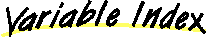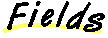`All Packages  Class Hierarchy  This Package  Previous  Next  Index`

```java.lang.Object
|
|
```

This probably doesn't belong in rsfF.solver, but MissingDataEquations is the only thing that currently uses it. Also, the name is probably not so good.

##complementmaskmaskZero

##SepMask(Rsf)
Create a mask which zeroes the components which are zero in maskRsf.SepMask(SepMask)

##copy()equals(Object)
Compares two Objects for equality.getComplement()
The complement of a SepMask, M, is the mask, C, which zeroes exactly those components which M does not zero.
Thus, C = I - M, and M.getComplement().getComplement() = M
(Here, I is the identity operator).toString()
Returns a string representation of the object.

##mask
``` protected boolean mask[]
```maskZero
``` protected boolean maskZero
```complement
``` protected SepMask complement
```

##SepMask
``` public SepMask(Rsf maskRsf)
```
Create a mask which zeroes the components which are zero in maskRsf.

Parameters:
maskRsf - may be subsequently changed without affecting "this".SepMask
``` protected SepMask(SepMask src)
```

##getComplement
``` public Mask getComplement()
```
The complement of a SepMask, M, is the mask, C, which zeroes exactly those components which M does not zero.
Thus, C = I - M, and M.getComplement().getComplement() = M
(Here, I is the identity operator).equals
``` public boolean equals(Object obj2)
```
Compares two Objects for equality.

Overrides:
equals in class SepSelfAdjointOperatorcopy
``` public JamObject copy()
```
Overrides:
copy in class SepSelfAdjointOperatortoString
``` public String toString()
```
Returns a string representation of the object.

Overrides:
toString in class Object

`All Packages  Class Hierarchy  This Package  Previous  Next  Index`